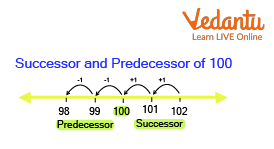Courses
Courses for Kids
Free study material
Offline Centres
More

# Successor and Predecessor NumberLast updated date: 25th Nov 2023
Total views: 141.6k
Views today: 4.41k## Introduction to Predecessor Number

“Honourable Mr. Narendra Modi is the 14th Prime Minister of the Republic of India. His predecessor was Dr. Manmohan Singh.”

We often see this sentence in our History or Social Studies books. But what does the term “predecessor” mean? Today, we will learn about successor and predecessor meanings and how to find them.

## Predecessors and Successors: What are They?

In Mathematics, the terms predecessor and successor are used almost always in context with another given whole number. In general terms, the predecessor or successor to a person or an object is that which comes before or after it, respectively. For example, ‘the king’s son was chosen to be his successor’ implies that after the king, his son would rule after him.

While on the other hand, "Pandit Jawaharlal Nehru was Lal Bahadur Shastri's predecessor as the prime minister of independent India." This implies that prior to Lal Bahadur Shastri's term as the Prime minister of Independent India, Pandit Jawaharlal Nehru was the prime minister in charge of the affairs of India and her people.

## What is Predecessor?

So what is the predecessor in mathematical terms? The predecessor is the number that “precedes” or comes before the given number. Simply put, the predecessor of a number is one less than it. Some examples of predecessors are-

The predecessor of 1 is 0.

The predecessor of 22 is 21.

The predecessor of 99 is 98.

For example, if “b” is the given value, then the predecessor of the given value (b) is the value “a”.

## How Do We Find the Predecessor?

The formula to derive the predecessor is \$(n-1)\$ where \$n\$ is the given value, and one is subtracted to show the predecessor of that number.

Hence the formula to derive the predecessor: Predecessor \$=n-1\$

## What is Successor?

Now that we have learned what the predecessor is, what is the successor in mathematics? The successor is the number that “succeeds” or comes after the given number. In simple terms, the successor of a number is one more than it. Some examples of successors are-

The successor of 1 is 2

The successor of 22 is 23

The successor of 99 is 100

For example, if the given value is 'b', then the successor of the given value (b) is the value 'c'.

## How Do We Find the Successor?

The formula to derive the successor is: ( n + 1 ) where n is the given value, and one is added to gain the successor of that number.

Hence the formula to derive the successor: Successor = n + 1Example of Successor and Predecessor

## Solved Examples

1. Find the predecessor for the following numbers:

1. 1000

2. 20

3. 345

Ans: The predecessors of the numbers are:

1. (1000-1) = 999

2. (20-1) = 19

3. (345-1) = 344

2. Find the successors for the following numbers:

1. 20

2. 9999

3. 4

Ans: The successors of the numbers are:

1. (20 + 1) = 21

2. (9999 + 1) = 10000

3. (4 + 1) = 5

## Practice Questions

1. If the given value is 99999, what is its successor? Upon deriving the answer, find the successor to the derived value as well.

2. The given value is 120. Find the predecessor of the given value. Also, find the predecessor of the answer.

1. Successor 1= 100000; Successor 2= 100001

2. Predecessor 1= 119; Successor 2= 118

## Summary

To sum up, what we have learned so far:

• The term predecessor refers to the number that precedes or comes before the given number.

• The term successor refers to the number that succeeds or comes after the given number.

• The formula to find the predecessor is: Predecessor = n - 1.

• The formula to find the successor is: Successor = n + 1.

• All whole numbers, upon using the formulae, may produce their respective successors and predecessors.

• A predecessor for the number zero is not possible, but its successor is 1.

## FAQs on Successor and Predecessor Number

1. What is the primary difference between the successor and the predecessor of a number?

The term predecessor refers to the number that precedes or comes before the given number whereas the successor of a number refers to the number that succeeds or comes after the given number. Generally, one would use the terms to describe the people who come before and after a particular given person, such as the predecessor of PM Indira Gandhi was PM Charan Singh while her successor was PM Rajiv Gandhi.

2. Why does the number zero not have a predecessor? Does it have a successor?

It is important to note that while all whole numbers may have predecessors and successors, the number zero (0) only has a successor and not a predecessor, i.e the successor of 0 is 1. There is no predecessor as that would be a negative number, which is not a whole number. Hence, a predecessor for the number zero is not possible.

3. Write the difference between successor and predecessor.

Differences between successor and predecessor are

 Successor Predecessor The number that comes just after a given number is called its successor. The number that comes just before a given number is called its predecessor. Examples:50 + 1 = 51; 29 + 1 = 30 Examples:50 - 1 = 49; 29 - 1 = 28 51 and 30 are the successors of 50 and 29, respectively. 49 and 28 are the predecessors of 50 and 29, respectively. Successor = Number + 1 Predecessor = Number - 1